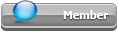## Featured Articles

Check out the latest featured articles.## File Library

Check out the latest downloads available in the File Library.0# Reactive Flash Drum

3 replies to this topic
Share this topic:
|

### #1chemical_teo

chemical_teo

Junior Member

•• Members
•• 18 posts

Posted 28 September 2018 - 02:05 AM

Hi all,

I have a conceptual question on something I have seen in my separation lecture. Indeed, we saw what a Flash Drum is and how to calculate the vapour or liquid flow rates or the liquid / vapour composition of each component of a system made up of two components. This is relatively easy since we only have do to a material balance and assume Raoult's law.

However, I saw somewhere that there were reactive flash (which we didn't mention yet in the lecture).

Basically, imagine we have two initial component A and B with molar fraction z_A and z_B in the feed (flow rate of the feed = F [mol/h]). Then, a reaction happens to form C and D. Let's suppose there is still some A and B left since the reaction is not complete. So, in the end we will get 4 species in the vapour phase (y_A, y_B, ...) and 4 species in the liquid phase (x_A, x_B, ...).

If I perform a material balance I get: F*z_i = L*x_i + V*y_i (as a non-reactive flash?!!) i = A, B, C and D. Assuming ideality, y_i = K_i * x_i . However, since I initially don't have any C (or D), z_C (or z_D) = 0. This would mean that x_C and y_C would be equal to 0. This obviously makes no sense...

Where is my mistake?

Thanks

### #2MrShorty

MrShorty

Gold Member

•• ChE Plus Subscriber
•• 458 posts

Posted 28 September 2018 - 10:33 AM

I think your mistake is trying to apply the same non-reactive material balance equation to a reactive system. Remember that the material balance equations are merely expressions of that fundamental law "the law of conservation of matter."

In a non-reactive system, I can treat A separate from B. I can say that the amount of A I get at the various outputs of the process must be the same as the amount of A I initially put into the process. In a reactive system, the amount of A at the outputs will not be the same as the amount of A I put in. I cannot really know how much A I will get out without also knowing how much B I put in, and knowing something about reaction rates and/or equilibrium constants and other properties of the reactive system. However, back to the fundamental law of conservation of matter, I can still say that the amount of A, B, C, and D at the outputs must be the same as the A and B I put in. The mathematics are necessarily more complex, because I need to know how the quantities of A, B, C, and D are interrelated, but the fundamental principle still applies.

### #3chemical_teo

chemical_teo

Junior Member

•• Members
•• 18 posts

Posted 29 September 2018 - 07:00 AM

Thank you very much. However, if we take a strong assumption saying that the reaction is assumed to reach equilibrium instantaneously, we could simply say we directly have A, B, C and D in the feed (taking the equilibrium molar fraction) and treat it as a classical flash drum?

### #4MrShorty

MrShorty

Gold Member

•• ChE Plus Subscriber
•• 458 posts

Posted 29 September 2018 - 10:38 PM

I do believe that would work.

### Similar Topics